GeeksforGeeks App
Open AppBrowser
Continue

# Python | Pandas MultiIndex.names

Python is a great language for doing data analysis, primarily because of the fantastic ecosystem of data-centric python packages. Pandas is one of those packages and makes importing and analyzing data much easier.

Pandas` MultiIndex.names` attribute returns the names of levels in the MultiIndex.

Syntax: MultiIndex.names

Example #1: Use `MultiIndex.names` attribute to find the names of the levels in the MultiIndex.

 `# importing pandas as pd``import` `pandas as pd`` ` `# Creating the array``array ``=` `[[``1``, ``2``, ``3``], [``'Sharon'``, ``'Nick'``, ``'Bailey'``]]`` ` `# Print the array``print``(array)`

Output :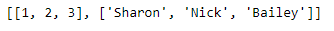Now let’s create the MultiIndex using this array

 `# Creating the MultiIndex``midx ``=` `pd.MultiIndex.from_arrays(array, names ``=``(``'Number'``, ``'Names'``))`` ` `# Print the MultiIndex``print``(midx)`

Output :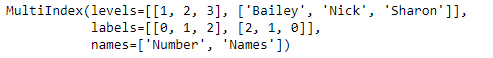Now we will find the names of all the levels in the MultiIndex.

 `# Print the names of the level in MultiIndex``midx.names`

Output :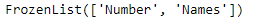As we can see in the output, midx has two levels and the name of the levels are ‘Number’ and ‘Names’.

Example #2: Use `MultiIndex.names` attribute to find the names of the levels in the given MultiIndex.

 `# importing pandas as pd``import` `pandas as pd`` ` `# Creating the array``array ``=``[[``1``, ``2``, ``3``], [``'Sharon'``, ``'Nick'``, ``'Bailey'``], ``           ``[``'Doctor'``, ``'Scientist'``, ``'Physicist'``]]`` ` `# Print the array``print``(array)`

Output :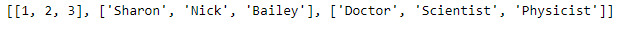Now let’s create the MultiIndex using this array

 `# Creating the MultiIndex``midx ``=` `pd.MultiIndex.from_arrays(array, ``   ``names ``=``(``'Ranking'``, ``'Names'``, ``'Profession'``))`` ` `# Print the MultiIndex``print``(midx)`

Output :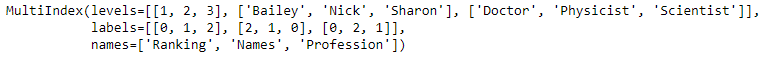Now we will find the names of all the levels in the MultiIndex.

 `# Print the names of the level in MultiIndex``midx.names`

Output :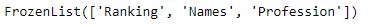As we can see in the output, midx has three levels and the name of the levels are ‘Number’, ‘Names’ and ‘Profession’.

My Personal Notes arrow_drop_up